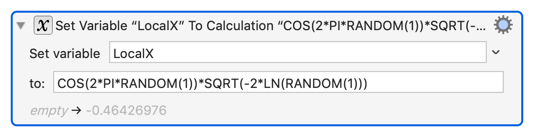# Mathematical enhancement to RAND

I have a good tip for people who use the RANDOM(X) function to generate natural processes (and this problem isn't specific to KM per se.) The problem that I have with the RANDOM function in most programming languages is that it generates an even/flat distribution of numbers between two values. Sometimes that's fine, but sometimes (when I'm trying to replicate natural processes with KM) I want the distribution to look more like a Normal Distribution.

So for most of the last 20 years I found a kludgy way to do that, usually something like this: (The PAUSE statement here is an example of where you might want this, if you are trying to emulate a human's "normal" delay to some process.)That routine generates a random number between 0 and 1 but it "favours" values around 0.5.

But by researching the topic enough I finally found a formula that generates a true Normal Distribution:There are two or three other formulas that can generate a Normal Distribution but this one doesn't require any IF statements so it's the most concise. It would be one of the first functions I would create if KM supported functions.

That function will generate a boundless random number centered around zero. However don't expect any large numbers above or below +/-5 unless you are willing to wait a million years. You are more likely to be hit by a falling airplane filled with chickens than get a large number like "5". (Actually I don't know enough math to tell you how long it will take to generate a number above 5. That was just a guess based on some sample data. I was never even able to get a "4". Nor do I know what the odds are of being hit by a plane load of chickens, but it's probably equally small.)

To show the idea in an image, I generated 999 random occurrences of this value using a KM macro and plotted it onto a bitmap. Here's a copy of that bitmap reduced to 75% size:

You can see visually that the distribution clusters in the middle but (you can probably sense that it) extends outwards with no actual outer limit.

Here's the distribution you would get with a (RANDOM(1)+RANDOM(1))/2 approach:

You can definitely infer from this diagram that it does have an outer limit. But I guess that's not as bad a result as I thought it would be. It does bear a visual resemblance to the Normal Distribution.

Maybe we can do a little better by using five RANDOM(1) calculations instead of two. So let's try the formula ((RANDOM(1)+RANDOM(1)+RANDOM(1)+RANDOM(1)+RANDOM(1))/5

That kinda looks like the Normal Distribution. Except it isn't. But to the human eye it's not a bad approximation. The clustering seems to approach the true Normal Distribution with 5 rather than with 2 values being added. However I've tried other values besides 5 and 2, like 22 and 55, and the results look worse to my eyes. However I am wondering if the limit as you approach infinity is the normal Distribution. It seems close when you use a multiplier to increase the scale, but I'm not sure what that multiplier would be.

You would think that the macro would be faster when using a few RANDOM(1) additions rather than using a COSINE, SQRT and LOG function. But in fact I timed the functions by themselves and found no measurable difference in speed. I guess modern CPUs can handle logarithms as fast as arithmetic.

In conclusion, if you want a random number that obeys a Normal Distribution rather than a flat distribution, use the formal above with the COSINE function in it, but if you want something close to a Normal Distribution with maximum limits, the multiple addition of about five RANDOM(1) approach is probably adequate. But if your macro is trying to simulate natural processes, the COSINE approach is required.

If anyone wants to see the code I used to create these graphs, I'd be happy to oblige, but that might be too much information for an opening post.

Any multi-degree mathematicians want to comment here? @CJK ?

1 Like